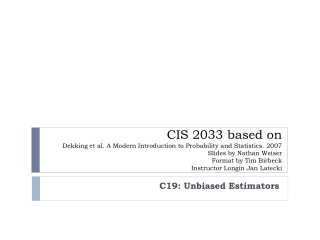DownloadDownload PresentationC19: Unbiased Estimators

# C19: Unbiased Estimators

Download Presentation## C19: Unbiased Estimators

- - - - - - - - - - - - - - - - - - - - - - - - - - - E N D - - - - - - - - - - - - - - - - - - - - - - - - - - -
##### Presentation Transcript

1. CIS 2033 based onDekking et al. A Modern Introduction to Probability and Statistics. 2007Slides by Nathan WeiserFormat by Tim Birbeck Instructor Longin Jan Latecki C19: Unbiased Estimators

2. 19.1 – Estimators • The parameters that determine the model distribution are called the model parameters • We focus on a situation where a parameter correspond to a feature of the model distribution that can be described by the model parameters themselves or by some function of the model parameters. This is known as the parameter of interest.

3. 19.1 – Estimators • ESTIMATE: an estimate is a value t that only depends on the dataset x1, x2,…,xn, i.e., t is some function that of the dataset only: • t = h(x1, x2,…,xn) • ESTIMATOR: Let t = h(x1, x2,…,xn) be an estimate based on the dataset x1, x2,…,xn.Then t is a realization of the random variable • T= h(X1, X2,…,Xn). • The random variable T is called an estimator. • Estimator refers to the method or device for estimation • Estimate refers to the actual value computed from a dataset

4. 19.2 Investigating the behavior of an estimator Estimating the probability p0 of zero arrivals, which is an unknown number between 0 and 1. Possible estimators:

5. 19.3 The Sampling Distribution and Unbiasedness • Desireable: E[S]=p0 • The Sampling Distribution: Let T= h(X1, X2,…,Xn) be an estimator based on a random sample X1, X2,…,Xn. The probability distribution of T is called the sampling distribution of T. • Sampling Distribution of S: Where Y is the number Xi equal to zero • Thus is follows that:

6. 19.3 The Sampling Distribution and Unbiasedness Definition: An estimator T is called an unbiased estimator for the parameter Ө,if E[T] = Ө Irrespective of the value of Ө. The difference E[T] – Ө is called the bias of T; if this difference is nonzero, then T is called biased

7. 19.3 The Sampling Distribution and Unbiasedness Definition: An estimator T is called an unbiased estimator for the parameter Ө,if E[T] = Ө Irrespective of the value of Ө. The difference E[T] – Ө is called the bias of T; if this difference is nonzero, then T is called biased

8. 19.4 Unbiased Estimators for Expectation and Variance Suppose X1, X2,…,Xnis a random sample from a distribution with finite expectation µ and finite variance σ2. Then: Is an unbiased estimator for µ and Is an unbiased estimator for σ2